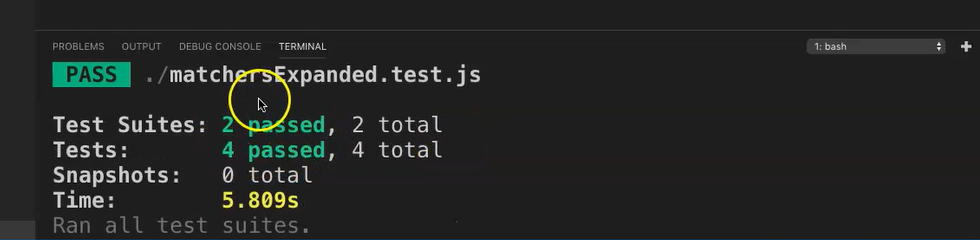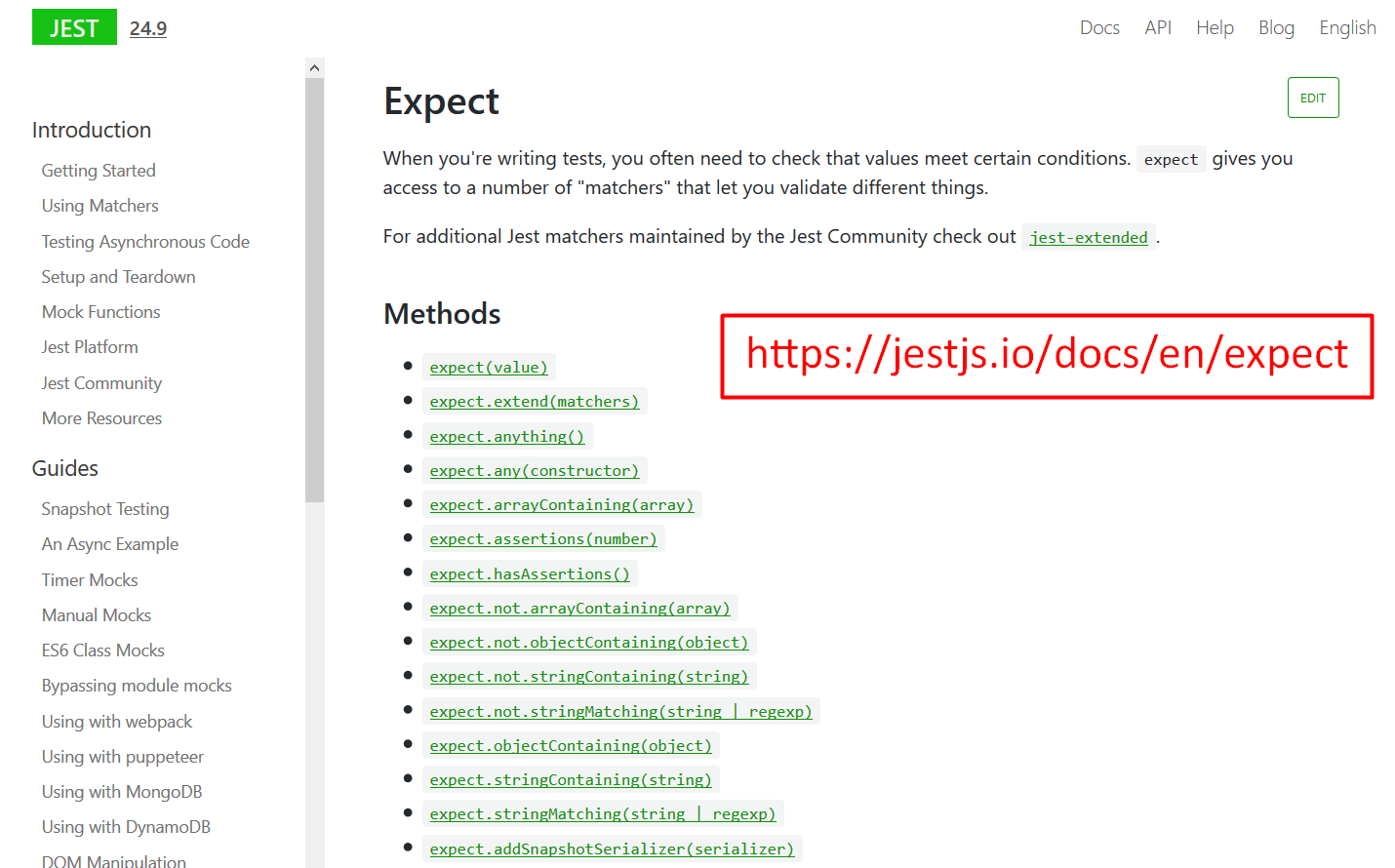Transcripted Summary

In the last chapter, we looked at what matchers were and looked specifically at the `.toBe` and `.toEqual` matchers.

In this chapter, we will look at some other matchers, which are very useful in Jest.

They include:

• String matchers
• Number matchers
• Truthiness matchers
• Array matchers

At that point, I will take you through to the official Jest documentation, where you can go through more matchers that would be useful to know.

### # String Matchers

You can easily test for certain String values by using regular expressions using the `.toMatch` matcher.

For example, if you want to check whether a certain word is contained in the larger word or sentence, then you can use it the following way.

Let's save the test once again and let's call it “matchersExpanded.test.js” (and remember our notation we need to add the `.test` or `.spec`.)

Let’s save this.

Let's say “there is pool in Liverpool” (and our JavaScript notation).

Then let's add the actual test in here [`expect('Liverpool')`].

And then let's say something like `.toMatch` and then, something similar to a regular expression.

``````test('there is pool in Liverpool',() => {
expect('Liverpool').toMatch(/pool/);
});
``````

Let's save this file now and then let's run this particular test.So, as you can see 2 test suites passed and 4 tests in total, those with the previous 3 that we had and this particular test.

Let's change this to not match now [“pol” instead of “pool”].

``````test('there is pool in Liverpool',() => {
expect('Liverpool').toMatch(/pol/);
});
``````

Let's save this and let's run it now.

As you can see, this now fails. If we look at our reason, expect “Liverpool” to match “pol” and obviously it doesn't find this match in there. And the test now fails.

### # Number Matchers

Let's move on to number matchers.

If we look at our previous tests using the `multiply` function and the “multiply.test”, we can expand on it by using some number type matchers.

Let's paste the code from the previous test in here, and now let's look at the `.toBe(6)`.

We can add more test on here by doing the following.

Let's copy this line, let's paste it here and then let's just change this to now say `.toBeGreaterThan`.

We can put 5 in here, which 6 is greater than 5. So, this test should work.

Let's paste another and maybe say `.toBeLessThan`.

And let's maybe say 7, save this.

Then let's paste one more and maybe let's say `.toBeLessThanOrEqual`.

My point here is just to take you through a few of the number matchers.

``````const multiply = require('./multiply');

describe('test multiply positive scenarios',() =>{
test('multiply 3*2 should equal to 6',() =>{
expect(multiply(3,2)).toBe(6);
expect(multiply(3,2)).toBeGreaterThan(5);
expect(multiply(3,2)).toBeLessThan(7);
expect(multiply(3,2)).toBeLessThanOrEqual(6);
});
})
``````

Let's save this and now let's run this particular test.

Great.

All our tests now pass, and you can see the “matchersExpanded.test.js” has actually passed.

### # Truthiness Matchers

Let's now move on to truthiness matchers.

Sometimes you would need to test some unconventional stuff like true or false, null or even undefined.

Let's look at a simple example which can also be found in the official Jest documentation.

``````test('null', () => {
const n = null;
expect(n).toBeNull();
expect(n).toBeDefined();
expect(n).not.toBeUndefined();
expect(n).not.toBeTruthy();
expect(n).toBeFalsy();
});
``````

We can now go through each line individually.

Let's write the `test` to test `null` and set `n` to `null`.

We `expect(n).toBeNull()`.

And then `expect(n).toBeDefined()` — and yes, this should work fine too as the “n” was defined above.

The next line is just a double negation and it is the same as `.toBeDefined` that we just spoke about.

The next two lines of matchers are related — the `Truthy` and `Falsy` matchers.

If something is said to be `Truthy`, then it is considered true if the value is considered true.

In this example, as `n` is set to be `null`, then this is a false value.

So, the lines, `.not.toBeTruthy` and `.toBeFalsy`, both should work as null is considered a false value.

Let's save this and run the test once more.

Great, we now have 6 passing tests as you can see in the “matchers.Expanded.test.js”.

### # Array Matchers

Finally, let's look at array's and the `.toContain` matcher.

Let's start with quickly defining an array like this. Let's say `const carStock = []` and let’s do an array.

And let's maybe say “BMW”. Let's add “Mercedes”; let's add maybe “Ferrari” in there.

Then finally, let's put a “Toyota” and close the array.

``````  const carStock = [
'BMW',
'Mercedes',
'Ferrari',
'Toyota',
];
``````

Let's just save that.

Now let's write the actual test, to test what's in the array.

``````test('that the car stock list has a Ferrari',() => {
expect(carStock).toContain('Ferrari');
});
``````

And let’s save that.

Now let's run the test. Enter, there we go.

That test past too, we now have 7 passing tests.

### # There are quite a few matchers one could still use.

You can check out the Jest official reference documentation for the matchers I've just mentioned.And a few others too, as you can see. There's some interesting ones in here too.

This then concludes the chapter on Jest matchers expanded.